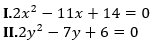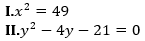Dear Aspirants,

### Quantitative Aptitude Quiz For SBI PO

Numerical Ability or Quantitative Aptitude Section has given heebie-jeebies to the aspirants when they appear for a banking examination. As the level of every other section is only getting complex and convoluted, there is no doubt that this section, too, makes your blood run cold. The questions asked in this section are calculative and very time-consuming. But once dealt with proper strategy, speed, and accuracy, this section can get you the maximum marks in the examination. Following is the Quantitative Aptitude quiz to help you practice with the best of latest pattern questions.

Q1. A milkman has 20 L of pure milk. He sells 5 L of it at profit of 20% and he mixes 5 L water in remaining quantity of milk. If he sells whole quantity of mixture at cost price then find his percentage profit?
25%
20%
24%
28%
30%

Q2. The sum of the radius and height of a cylinder is 18m. The total surface area of the cylinder is 792 m², what is the volume of the cylinder ? (in m³)
1848
1694
1716
1724
1440

Q3. In a 90 litre mixture of milk and water, percentage of water is only 30%. The milkman gave 18 litres of this mixture to a customer and then added 18 litres of water to the remaining mixture. What is the percentage of milk in the final mixture ?
64%
48%
52%
68%
56%

Q4. If the area of a circle is 616 cm², what would be the total surface area of a hemisphere having the same radius as the circle ?
1848 cm²
1648 cm²
2218 cm²
1808 cm²
1765cm²

Q5. There are two mixtures of honey and water, the quantity of honey in them being 40% and 75% of the mixture respectively. If 5 gallons of the first are mixed with 8 gallons of the second, what will be the ratio of honey to water in the new mixture ?
11 : 2
8 : 5
9 : 11
2 : 11
3 : 22

Directions (6-10): There are total five departments in a company. There are total 90 employees in Finance department which is 25% of total employees in the company. 2/9 of the total employees of the company are working in HR department. Employees working in Sales department is 25% more than that in HR department. Ratio between employees working in Security and Housing department is 4 : 5.

Q6. Find number of employees working in HR department is what percent more than number of employees working in Security department?
250%
200%
150%
100%
50%

Q7. Find the average number of employees working in Sales, Finance and Housing department?
60
70
80
90
100

Q8. Number of employees in Housing department is how much more than number of employees in Security department?
10
20
30
40
50

Q9. In Security department, 40% are female employees then find total male employees working in Security department?
16
40
32
8
24

Q10. Ratio between total number of male and female employees in HR department is 2 : 3. Find total number of female employees working in HR department?
32
48
64
40
56

Directions (11-15): In each of the following questions two equations are given. Solve the equations and give answer—

Q11.If x < y
If x ≤ y
x = y or relationship between x and y cannot be established.
If x ≥ y
If x > y

Q12.If x < y
If x ≤ y
x = y or relationship between x and y cannot be established.
If x ≥ y
If x > y

Q13.If x < y
If x ≤ y
x = y or relationship between x and y cannot be established.
If x ≥ y
If x > y

Q14.If x < y
If x ≤ y
x = y or relationship between x and y cannot be established.
If x ≥ y
If x > y

Q15.If x < y
If x ≤ y
x = y or relationship between x and y cannot be established.
If x ≥ y
If x > y

You May also like to Read: